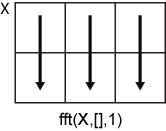# fft

## 语法

Y = fft(X)
Y = fft(X,n)
Y = fft(X,n,dim)

## 说明

Y = fft(X) 用快速傅里叶变换 (FFT) 算法计算 X 的离散傅里叶变换 (DFT)。 如果 X 是向量，则 fft(X) 返回该向量的傅里叶变换。如果 X 是矩阵，则 fft(X) 将 X 的各列视为向量，并返回每列的傅里叶变换。如果 X 是一个多维数组，则 fft(X) 将沿大小不等于 1 的第一个数组维度的值视为向量，并返回每个向量的傅里叶变换。 

Y = fft(X,n) 返回 n 点 DFT。如果未指定任何值，则 Y 的大小与 X 相同。 如果 X 是向量且 X 的长度小于 n，则为 X 补上尾零以达到长度 n。如果 X 是向量且 X 的长度大于 n，则对 X 进行截断以达到长度 n。如果 X 是矩阵，则每列的处理与在向量情况下相同。如果 X 为多维数组，则大小不等于 1 的第一个数组维度的处理与在向量情况下相同。 

Y = fft(X,n,dim) 返回沿维度 dim 的傅里叶变换。例如，如果 X 是矩阵，则 fft(X,n,2) 返回每行的 n 点傅里叶变换。

## 示例

Fs = 1000; % Sampling frequency T = 1/Fs; % Sampling period L = 1500; % Length of signal t = (0:L-1)*T; % Time vector

S = 0.7*sin(2*pi*50*t) + sin(2*pi*120*t);

X = S + 2*randn(size(t));

plot(1000*t(1:50),X(1:50)) title("Signal Corrupted with Zero-Mean Random Noise") xlabel("t (milliseconds)") ylabel("X(t)")Y = fft(X);

P2 = abs(Y/L); P1 = P2(1:L/2+1); P1(2:end-1) = 2*P1(2:end-1);

f = Fs*(0:(L/2))/L; plot(f,P1) title("Single-Sided Amplitude Spectrum of X(t)") xlabel("f (Hz)") ylabel("|P1(f)|")Y = fft(S); P2 = abs(Y/L); P1 = P2(1:L/2+1); P1(2:end-1) = 2*P1(2:end-1); plot(f,P1) title("Single-Sided Amplitude Spectrum of S(t)") xlabel("f (Hz)") ylabel("|P1(f)|")Fs = 44100; % Sampling frequency T = 1/Fs; % Sampling period t = -0.5:T:0.5; % Time vector L = length(t); % Signal length X = 1/(0.4*sqrt(2*pi))*(exp(-t.^2/(2*(0.1*1e-3)^2)));

plot(t,X) title("Gaussian Pulse in Time Domain") xlabel("Time (t)") ylabel("X(t)") axis([-1e-3 1e-3 0 1.1])fft 的执行时间取决于变换的长度。仅具有小质因数的变换长度比那些具有大质因数的变换长度的执行时间要快得多。

n = 2^nextpow2(L);

Y = fft(X,n);

f = Fs*(0:(n/2))/n; P = abs(Y/n).^2; plot(f,P(1:n/2+1)) title("Gaussian Pulse in Frequency Domain") xlabel("f (Hz)") ylabel("|P(f)|^2")Fs = 1000; % Sampling frequency T = 1/Fs; % Sampling period L = 1000; % Length of signal t = (0:L-1)*T; % Time vector

x1 = cos(2*pi*50*t); % First row wave x2 = cos(2*pi*150*t); % Second row wave x3 = cos(2*pi*300*t); % Third row wave X = [x1; x2; x3];

for i = 1:3 subplot(3,1,i) plot(t(1:100),X(i,1:100)) title("Row " + num2str(i) + " in the Time Domain") enddim = 2;

Y = fft(X,L,dim);

P2 = abs(Y/L); P1 = P2(:,1:L/2+1); P1(:,2:end-1) = 2*P1(:,2:end-1);

for i=1:3 subplot(3,1,i) plot(0:(Fs/L):(Fs/2-Fs/L),P1(i,1:L/2)) title("Row " + num2str(i) + " in the Frequency Domain") endFs = 100; t = 0:1/Fs:1-1/Fs; x = cos(2*pi*15*t - pi/4) + cos(2*pi*40*t + pi/2);

y = fft(x); z = fftshift(y); ly = length(y); f = (-ly/2:ly/2-1)/ly*Fs; stem(f,abs(z)) title("Double-Sided Amplitude Spectrum of x(t)") xlabel("Frequency (Hz)") ylabel("|y|") gridtol = 1e-6; z(abs(z) < tol) = 0; theta = angle(z); stem(f,theta/pi) title("Phase Spectrum of x(t)") xlabel("Frequency (Hz)") ylabel("Phase/\pi") gridFs = 80; T = 1/Fs; L = 65; t = (0:L-1)*T;

X = 3*cos(2*pi*2*t) + 2*cos(2*pi*4*t) + sin(2*pi*6*t);

plot(t,X) title("Signal superposition in time domain") xlabel("t (ms)") ylabel("X(t)")Y = fft(X);

f = Fs*(0:(L-1)/2)/L; P2 = abs(Y/L); P1 = P2(1:(L+1)/2); P1(2:end) = 2*P1(2:end);

plot(f,P1,"-o") title("Single-Sided Spectrum of Original Signal") xlabel("f (Hz)") ylabel("|P1(f)|")n = 2^nextpow2(L); Y = fft(X,n);

f = Fs*(0:(n/2))/n; P2 = abs(Y/L); P1 = P2(1:n/2+1); P1(2:end-1) = 2*P1(2:end-1);

plot(f,P1,"-o") title("Single-Sided Spectrum of Padded Signal") xlabel("f (Hz)") ylabel("|P1(f)|")## 输入参数

• fft(X,[],1) 沿 X 的各列进行运算，并返回每列的傅里叶变换。• fft(X,[],2) 沿 X 的各行进行运算，并返回每行的傅里叶变换。## 输出参数

Y 的大小如下：

• 对于 Y = fft(X)Y = fft(X,[],dim)Y 的大小等于 X 的大小。

• 对于 Y = fft(X,n,dim)size(Y,dim) 的值等于 n，而所有其他维度的大小保持与在 X 中相同。

## 详细信息

### 向量的离散傅里叶变换

Y = fft(X)X = ifft(Y) 分别实现傅里叶变换和傅里叶逆变换。对于长度为 nXY，这些变换定义如下：

$\begin{array}{l}Y\left(k\right)=\sum _{j=1}^{n}X\left(j\right)\text{\hspace{0.17em}}{W}_{n}^{\left(j-1\right)\text{​}\left(k-1\right)}\\ X\left(j\right)=\frac{1}{n}\sum _{k=1}^{n}Y\left(k\right)\text{\hspace{0.17em}}{W}_{n}{}^{-\left(j-1\right)\text{​}\left(k-1\right)},\end{array}$

${W}_{n}={e}^{\left(-2\pi i\right)/n}$

## 提示

• fft 的执行时间取决于变换的长度。仅具有小质因数（不大于 7）的变换长度的执行时间明显快于本身是质数或具有较大质因数的变换长度的执行时间。

• 对于大多数 n 值，实数输入的 DFT 需要的计算时间大致是复数输入的 DFT 计算时间的一半。但是，当 n 有较大的质因数时，速度很少有差别或没有差别。

• 使用工具函数 fftw 可能会提高 fft 的速度。此函数控制用于计算特殊大小和维度的 FFT 算法优化。

## 算法

FFT 函数（fftfft2fftnifftifft2ifftn）基于一个称为 FFTW   的库。

 Frigo, M., and S. G. Johnson. “FFTW: An Adaptive Software Architecture for the FFT.” Proceedings of the International Conference on Acoustics, Speech, and Signal Processing. Vol. 3, 1998, pp. 1381-1384.